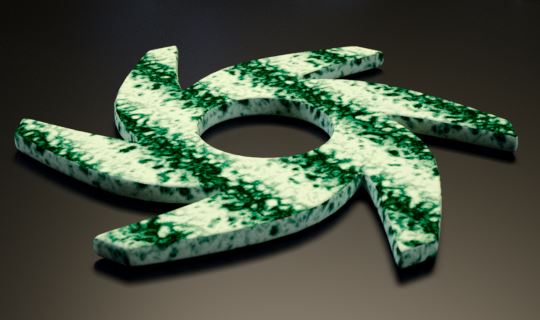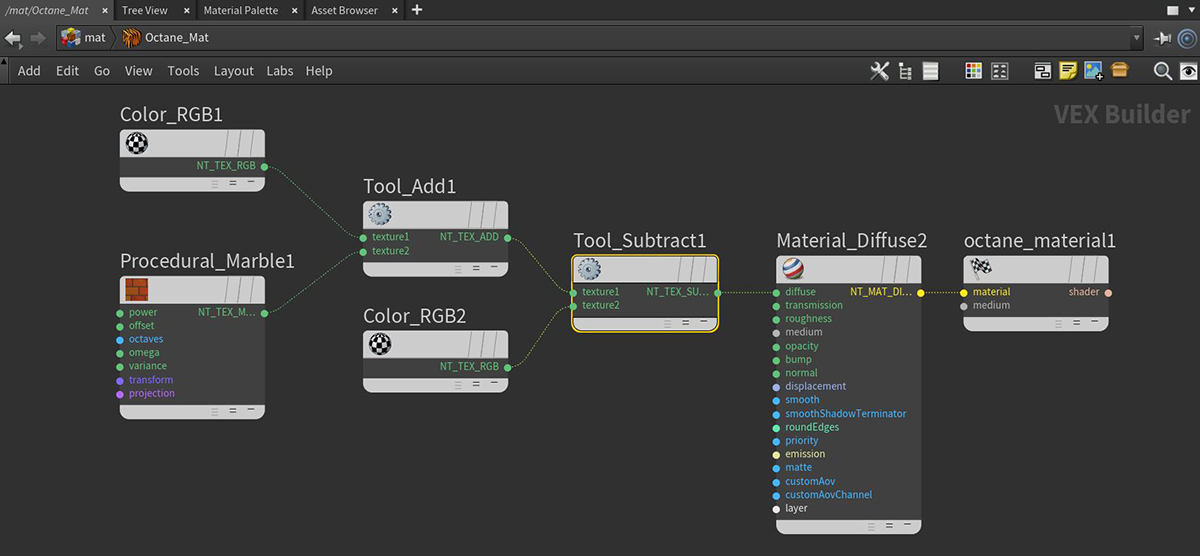# Tool Subtract

The Subtract node subtracts pixel values of one incoming texture with the pixel values of the other incoming texture.

In Figure 1, a Marble texture is tinted red using the Add node. The Marble texture is then blended with an RGBcolor node (also set to red) using the Subtract node. The result is a greenish/blue color due to the reds cancelling or subtracting each other.Figure 1: Using the Subtract node to mix a Marble texture with two RGB color nodesFigure 2: Subtract node network used for the rendering in Figure 1